Drop a Query

# Data Manipulation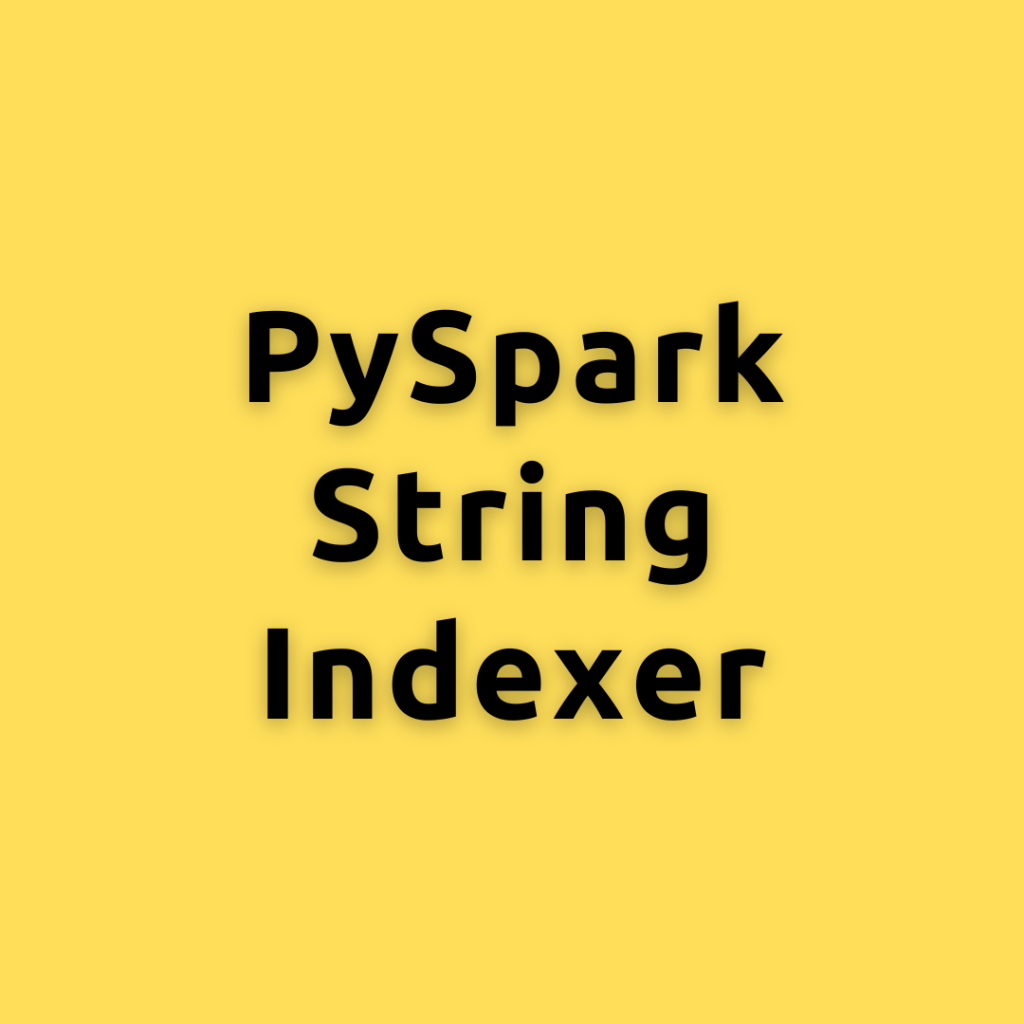## PySpark StringIndexer – A Comprehensive Guide to master PySpark StringIndexer

Deep understanding of PySpark’s StringIndexe, how it works, and how to effectively use it in your PySpark workflow Machine learning practitioners often encounter categorical data that needs to be transformed into a numerical format. We will delve into PySpark’s StringIndexer, an essential feature that converts categorical string columns into numerical indices. This guide will provide …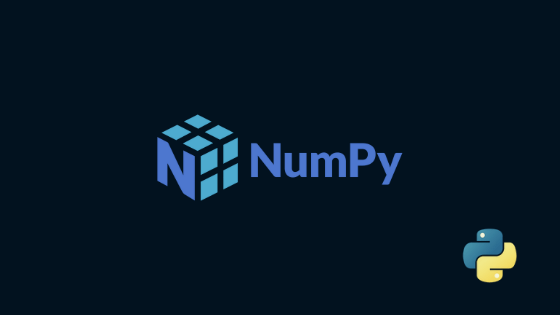## How to use Numpy Random Function in Python

How to use numpy.random.rand() function ? numpy.random.rand() function is used to generate random float values from an uniform distribution over [0,1). These values can be extracted as a single value or in arrays of any dimension. In this article, you will learn about various use cases of this function. Structural overview of numpy.random.rand() Syntax: numpy.random.rand(d0, …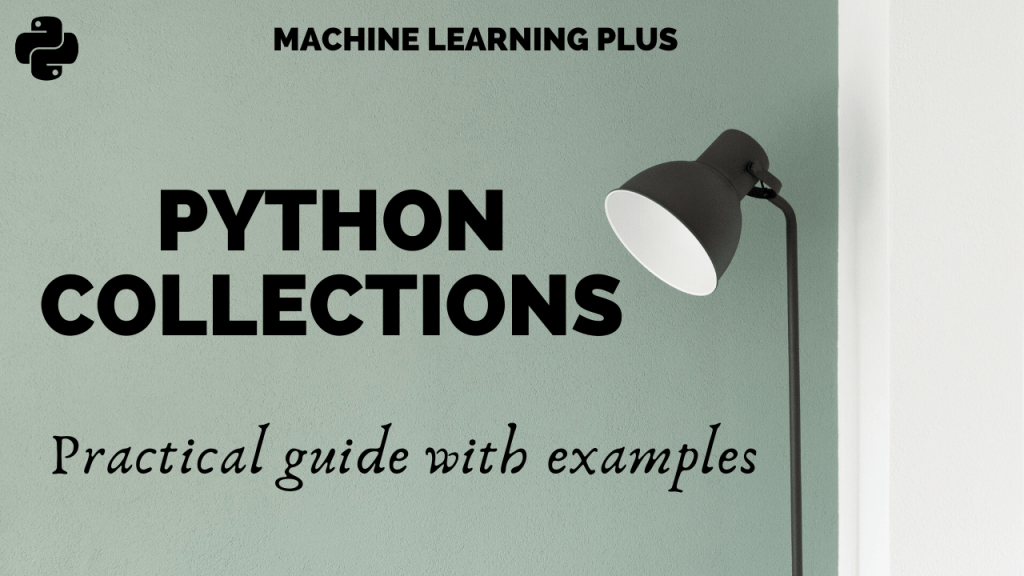## Python Collections – An Introductory Guide

Collections is a built-in python module that provides useful container datatypes. Container datatypes allow us to store and access values in a convenient way. Generally, you would have used lists, tuples, and dictionaries. But, while dealing with structured data we need smarter objects. In this article, I will walk you through the different data structures …## DataFrames in Julia

DataFrame is a 2 dimensional mutable data structure, that is used for handling tabular data. Unlike Arrays and Matrices, a DataFrame can hold columns of different data types The DataFrames package in Julia provides the DataFrame object which is used to hold and manipulate tabular data in a flexible and convenient way. It is quite …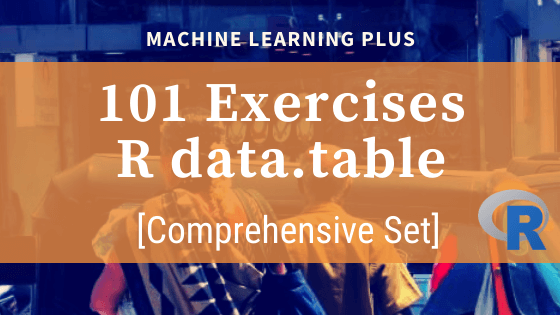## 101 R data.table Exercises

The data.table package in R is super fast when it comes to handling data. It has a syntax that reduces keystrokes while making R code easier to read. These set of exercises are designed to help you to oil your data brain through solving data manipulation exercises. Related post: 101 Python datatable Exercises (pydatatable) 101 …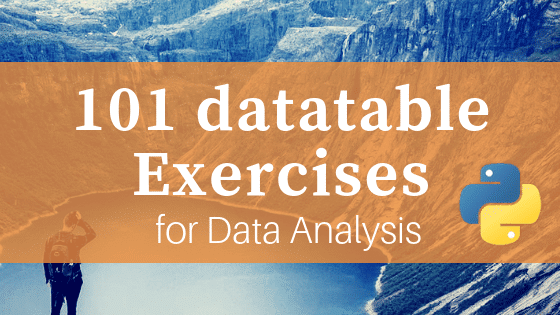## 101 Python datatable Exercises (pydatatable)

Python datatable is the newest package for data manipulation and analysis in Python. It carries the spirit of R’s data.table with similar syntax. It is super fast, much faster than pandas and has the ability to work with out-of-memory data. Looking at the performance it is on path to become a must-use package for data …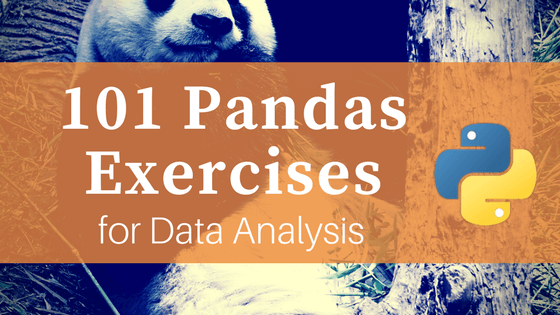## 101 Pandas Exercises for Data Analysis

101 python pandas exercises are designed to challenge your logical muscle and to help internalize data manipulation with python’s favorite package for data analysis. The questions are of 3 levels of difficulties with L1 being the easiest to L3 being the hardest. 101 Pandas Exercises. Photo by Chester Ho. You might also like to practice …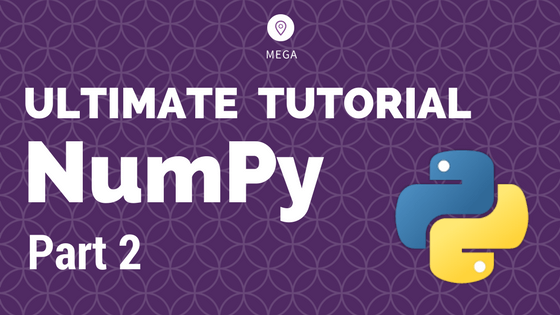## Numpy Tutorial Part 2 – Vital Functions for Data Analysis

Numpy is the core package for data analysis and scientific computing in python. This is part 2 of a mega numpy tutorial. In this part, I go into the details of the advanced features of numpy that are essential for data analysis and manipulations. Introduction How to get index locations that satisfy a given condition …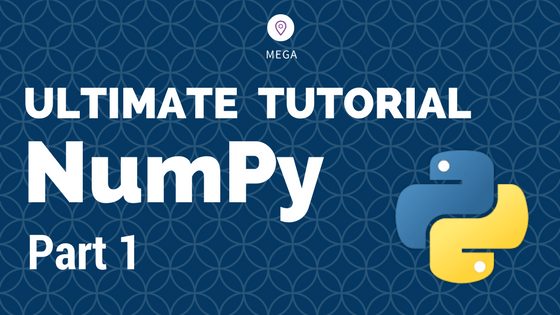## Numpy Tutorial – Your first numpy guide to build python coding foundations

This is part 1 of the numpy tutorial covering all the core aspects of performing data manipulation and analysis with numpy’s ndarrays. Numpy is the most basic and a powerful package for scientific computing and data manipulation in python. Numpy Tutorial Part 1: Introduction to Arrays. Photo by Bryce Canyon. Also Read: Numpy Tutorial – …Course Preview

## Machine Learning A-Z™: Hands-On Python & R In Data Science

### Free Sample Videos:#### Machine Learning A-Z™: Hands-On Python & R In Data Science#### Machine Learning A-Z™: Hands-On Python & R In Data Science#### Machine Learning A-Z™: Hands-On Python & R In Data Science#### Machine Learning A-Z™: Hands-On Python & R In Data Science